# Dimensionality reduction for input data

Last updated on：2 years ago

Dimensionality reduction plays an essential part in speeding up learning processes.

# Basic ideas

Data compression

eg. reduce data from 2D to 1D
eg. reduce data from 3D to 2D

Data visualization

1D, 2D, 3D

# Principal component analysis/PCA

Try to find a lower dimensional surface, so that the sum value of the square of these group line segment is small.

Reduce from n-dimension to k-dimension: find k vector $u^{(1)}, u^{(2)}, …, u^{(K)}$ onto which to project the data to minimize the projection error.

## PCA is not linear regression

Linear regression vs. PCA

$X\to y$ VS. treated $x_1, x_2, …, x_m$ equally.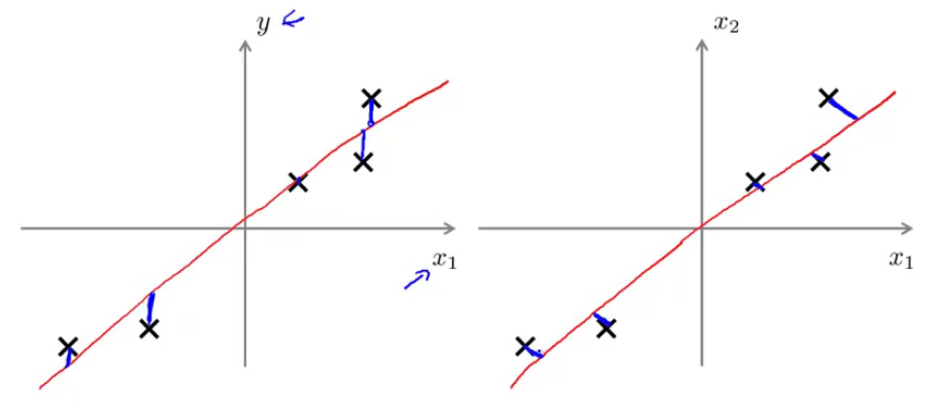## Principal component analysis algorithm

• Pre-processing (feature scaling/mean normalization):

Replace each $x_j^{(i)}$ with $x_j - \mu_j$
Scale features to have comparable range of values.

• Compute covariance matrix: $\sum = \frac{1}{m} \sum_{i=1}^n (x^{(i)}) (x^{(j)}) ^T$

(satisfy symmetric positive definite)

• Compute eigenvectors of matrix $\sum$:

[U, S, V] = svd(Sigma);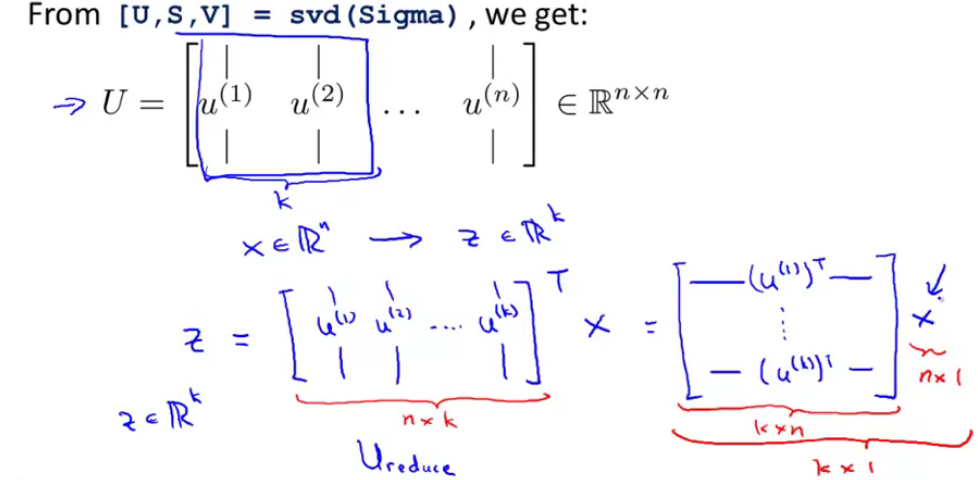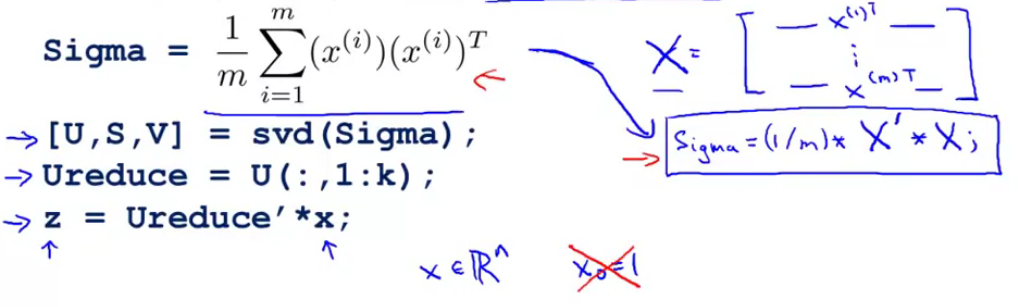## Reconstruction from the compressed representation

$$z = U^T_{reduce} x$$
$$X_{approx}^{(1)} = U_{reduce} z^{(1)}$$
$$R^n = (n\times k) (k\times 1)$$

## Choosing k the number of principal components

• Average squared projection error: $$\frac{1}{m} \sum^m_{i=1} ||x^{(i)} - x^{(i)}_{approx}|| ^2$$
• Total variation in the data: $\frac{1}{m} \sum^m_{i=1} ||x^{(i)}|| ^2$

Typically, choose k to be smallest value so that
$$\frac{\frac{1}{m} \sum^m_{i=1} ||x^{(i)} - x^{(i)}{approx}|| ^2}{\frac{1}{m} \sum^m{i=1} ||x^{(i)}|| ^2} <= 0.01$$
99% of variance is retained.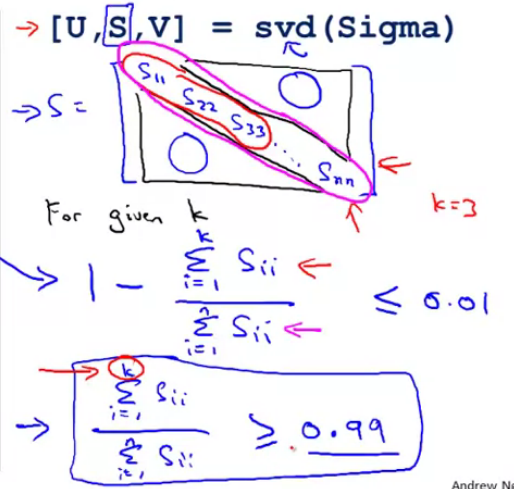• Extract inputs from an unlabelled dataset
• New training set

Note: mapping $x \to z$ should be defined by running PCA only on the training set. This mapping can be applied as well to the examples $x_cv$ and $x_test$ in the cross validation and test sets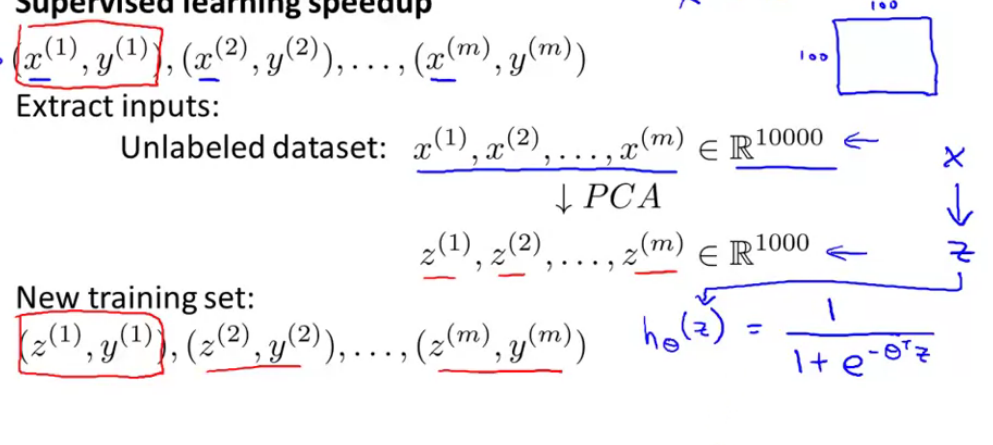# Design of ML system

• Get training set
• Run PCA to reduce $x^{(i)}$ in dimension to get $z^{(i)}$
• Train logistic regression on
• Test on test set: map $$x_{test}^{(i)} \to z^{(i)}_{test}$$

Before implementing PCA, try running whatever you want to do with the original/raw data $x^{(i)}$ first . only if that doesn’t do what you want, then implement PCA and consider using $z^{(i)}$

# Reference

 Andrew NG, Machine learning